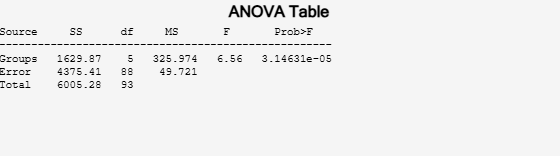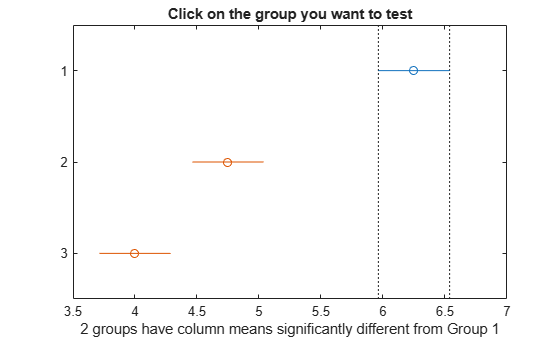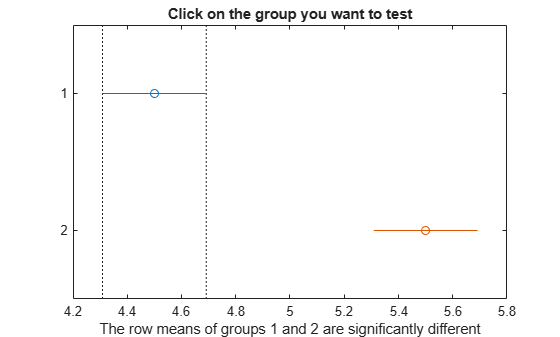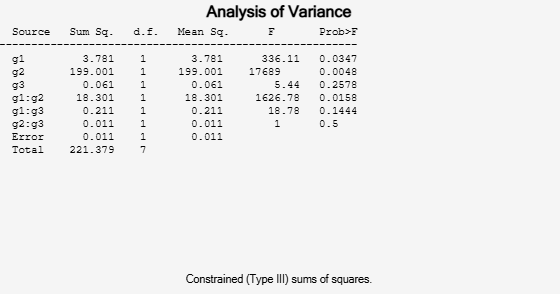Documentation

# multcompare

Multiple comparison test

## Syntax

``c = multcompare(stats)``
``c = multcompare(stats,Name,Value)``
``````[c,m] = multcompare(___)``````
``````[c,m,h] = multcompare(___)``````
``````[c,m,h,gnames] = multcompare(___)``````

## Description

example

````c = multcompare(stats)` returns a matrix `c` of the pairwise comparison results from a multiple comparison test using the information contained in the `stats` structure. `multcompare` also displays an interactive graph of the estimates and comparison intervals. Each group mean is represented by a symbol, and the interval is represented by a line extending out from the symbol. Two group means are significantly different if their intervals are disjoint; they are not significantly different if their intervals overlap. If you use your mouse to select any group, then the graph will highlight all other groups that are significantly different, if any.```

example

````c = multcompare(stats,Name,Value)` returns a matrix of pairwise comparison results, `c`, using additional options specified by one or more `Name,Value` pair arguments. For example, you can specify the confidence interval, or the type of critical value to use in the multiple comparison.```
``````[c,m] = multcompare(___)``` also returns a matrix, `m`, which contains estimated values of the means (or whatever statistics are being compared) for each group and the corresponding standard errors. You can use any of the previous syntaxes.```
``````[c,m,h] = multcompare(___)``` also returns a handle, `h`, to the comparison graph. ```

example

``````[c,m,h,gnames] = multcompare(___)``` also returns a cell array, `gnames`, which contains the names of the groups.```

## Examples

collapse all

Load the sample data.

`load carsmall`

Perform a one-way analysis of variance (ANOVA) to see if there is any difference between the mileage of the cars by origin.

`[p,t,stats] = anova1(MPG,Origin,'off');`

Perform a multiple comparison of the group means.

`[c,m,h,nms] = multcompare(stats);``multcompare` displays the estimates with comparison intervals around them. You can click the graphs of each country to compare its mean to those of other countries.

Now display the mean estimates and the standard errors with the corresponding group names.

`[nms num2cell(m)]`
```ans = 6x3 cell array {'USA' } {[21.1328]} {[0.8814]} {'Japan' } {[31.8000]} {[1.8206]} {'Germany'} {[28.4444]} {[2.3504]} {'France' } {[23.6667]} {[4.0711]} {'Sweden' } {[22.5000]} {[4.9860]} {'Italy' } {[ 28]} {[7.0513]} ```

Load the sample data.

```load popcorn popcorn```
```popcorn = 6×3 5.5000 4.5000 3.5000 5.5000 4.5000 4.0000 6.0000 4.0000 3.0000 6.5000 5.0000 4.0000 7.0000 5.5000 5.0000 7.0000 5.0000 4.5000 ```

The data is from a study of popcorn brands and popper types (Hogg 1987). The columns of the matrix `popcorn` are brands (Gourmet, National, and Generic). The rows are popper types oil and air. In the study, researchers popped a batch of each brand three times with each popper. The values are the yield in cups of popped popcorn.

Perform a two-way ANOVA. Also compute the statistics that you need to perform a multiple comparison test on the main effects.

`[~,~,stats] = anova2(popcorn,3,'off')`
```stats = struct with fields: source: 'anova2' sigmasq: 0.1389 colmeans: [6.2500 4.7500 4] coln: 6 rowmeans: [4.5000 5.5000] rown: 9 inter: 1 pval: 0.7462 df: 12 ```

The `stats` structure includes

• The mean squared error (`sigmasq`)

• The estimates of the mean yield for each popcorn brand (`colmeans`)

• The number of observations for each popcorn brand (`coln`)

• The estimate of the mean yield for each popper type (`rowmeans`)

• The number of observations for each popper type (`rown`)

• The number of interactions (`inter`)

• The p-value that shows the significance level of the interaction term (`pval`)

• The error degrees of freedom (`df`).

Perform a multiple comparison test to see if the popcorn yield differs between pairs of popcorn brands (columns).

`c = multcompare(stats)`
```Note: Your model includes an interaction term. A test of main effects can be difficult to interpret when the model includes interactions. ``````c = 3×6 1.0000 2.0000 0.9260 1.5000 2.0740 0.0000 1.0000 3.0000 1.6760 2.2500 2.8240 0.0000 2.0000 3.0000 0.1760 0.7500 1.3240 0.0116 ```

The first two columns of `c` show the groups that are compared. The fourth column shows the difference between the estimated group means. The third and fifth columns show the lower and upper limits for 95% confidence intervals for the true mean difference. The sixth column contains the p-value for a hypothesis test that the corresponding mean difference is equal to zero. All p-values (0, 0, and 0.0116) are very small, which indicates that the popcorn yield differs across all three brands.

The figure shows the multiple comparison of the means. By default, the group 1 mean is highlighted and the comparison interval is in blue. Because the comparison intervals for the other two groups do not intersect with the intervals for the group 1 mean, they are highlighted in red. This lack of intersection indicates that both means are different than group 1 mean. Select other group means to confirm that all group means are significantly different from each other.

Perform a multiple comparison test to see the popcorn yield differs between the two popper types (rows).

`c = multcompare(stats,'Estimate','row')`
```Note: Your model includes an interaction term. A test of main effects can be difficult to interpret when the model includes interactions. ``````c = 1×6 1.0000 2.0000 -1.3828 -1.0000 -0.6172 0.0001 ```

The small p-value of 0.0001 indicates that the popcorn yield differs between the two popper types (air and oil). The figure shows the same results. The disjoint comparison intervals indicate that the group means are significantly different from each other.

Load the sample data.

```y = [52.7 57.5 45.9 44.5 53.0 57.0 45.9 44.0]'; g1 = [1 2 1 2 1 2 1 2]; g2 = {'hi';'hi';'lo';'lo';'hi';'hi';'lo';'lo'}; g3 = {'may';'may';'may';'may';'june';'june';'june';'june'};```

`y` is the response vector and `g1`, `g2`, and `g3` are the grouping variables (factors). Each factor has two levels, and every observation in `y` is identified by a combination of factor levels. For example, observation `y(1)` is associated with level 1 of factor `g1`, level `'hi'` of factor `g2`, and level `'may'` of factor `g3`. Similarly, observation `y(6)` is associated with level 2 of factor `g1`, level `'hi'` of factor `g2`, and level `'june'` of factor `g3`.

Test if the response is the same for all factor levels. Also compute the statistics required for multiple comparison tests.

```[~,~,stats] = anovan(y,{g1 g2 g3},'model','interaction',... 'varnames',{'g1','g2','g3'});```The p-value of 0.2578 indicates that the mean responses for levels `'may'` and `'june'` of factor `g3` are not significantly different. The p-value of 0.0347 indicates that the mean responses for levels `1` and `2` of factor `g1` are significantly different. Similarly, the p-value of 0.0048 indicates that the mean responses for levels `'hi'` and `'lo'` of factor `g2` are significantly different.

Perform multiple comparison tests to find out which groups of the factors `g1` and `g2` are significantly different.

`results = multcompare(stats,'Dimension',[1 2])````results = 6×6 1.0000 2.0000 -6.8604 -4.4000 -1.9396 0.0280 1.0000 3.0000 4.4896 6.9500 9.4104 0.0177 1.0000 4.0000 6.1396 8.6000 11.0604 0.0143 2.0000 3.0000 8.8896 11.3500 13.8104 0.0108 2.0000 4.0000 10.5396 13.0000 15.4604 0.0095 3.0000 4.0000 -0.8104 1.6500 4.1104 0.0745 ```

`multcompare` compares the combinations of groups (levels) of the two grouping variables, `g1` and `g2`. In the `results` matrix, the number 1 corresponds to the combination of level `1` of `g1` and level `hi` of `g2`, the number 2 corresponds to the combination of level `2` of `g1` and level `hi` of `g2`. Similarly, the number 3 corresponds to the combination of level `1` of `g1` and level `lo` of `g2`, and the number 4 corresponds to the combination of level `2` of `g1` and level `lo` of `g2`. The last column of the matrix contains the p-values.

For example, the first row of the matrix shows that the combination of level `1` of `g1` and level `hi` of `g2` has the same mean response values as the combination of level `2` of `g1` and level `hi` of `g2`. The p-value corresponding to this test is 0.0280, which indicates that the mean responses are significantly different. You can also see this result in the figure. The blue bar shows the comparison interval for the mean response for the combination of level `1` of `g1` and level `hi` of `g2`. The red bars are the comparison intervals for the mean response for other group combinations. None of the red bars overlap with the blue bar, which means the mean response for the combination of level `1` of `g1` and level `hi` of `g2` is significantly different from the mean response for other group combinations.

You can test the other groups by clicking on the corresponding comparison interval for the group. The bar you click on turns to blue. The bars for the groups that are significantly different are red. The bars for the groups that are not significantly different are gray. For example, if you click on the comparison interval for the combination of level `1` of `g1` and level `lo` of `g2`, the comparison interval for the combination of level `2` of `g1` and level `lo` of `g2` overlaps, and is therefore gray. Conversely, the other comparison intervals are red, indicating significant difference.

## Input Arguments

collapse all

Test data, specified as a structure. You can create a structure using one of the following functions:

`multcompare` does not support multiple comparisons using `anovan` output for a model that includes random or nested effects. The calculations for a random effects model produce a warning that all effects are treated as fixed. Nested models are not accepted.

Data Types: `struct`

### Name-Value Pair Arguments

Specify optional comma-separated pairs of `Name,Value` arguments. `Name` is the argument name and `Value` is the corresponding value. `Name` must appear inside quotes. You can specify several name and value pair arguments in any order as `Name1,Value1,...,NameN,ValueN`.

Example: `'Alpha',0.01,'CType','bonferroni','Display','off'` computes the Bonferroni critical values, conducts the hypothesis tests at the 1% significance level, and omits the interactive display.

Significance level of the multiple comparison test, specified as the comma-separated pair consisting of `'Alpha'` and a scalar value in the range (0,1). The value specified for `'Alpha'` determines the 100 × (1 – α) confidence levels of the intervals returned in the matrix `c` and in the figure.

Example: `'Alpha',0.01`

Data Types: `single` | `double`

Type of critical value to use for the multiple comparison, specified as the comma-separated pair consisting of `'CType'` and one of the following.

ValueDescription
`'tukey-kramer'` or `'hsd'`

Tukey's honest significant difference criterion

`'bonferroni'`

Bonferroni method

`'dunn-sidak'`

Dunn and Sidák’s approach

`'lsd'`

Fisher's least significant difference procedure

`'scheffe'`

Scheffé's S procedure

Example: `'CType','bonferroni'`

Display toggle, specified as the comma-separated pair consisting of `'Display'` and either `'on'` or `'off'`. If you specify `'on'`, then `multcompare` displays a graph of the estimates and their comparison intervals. If you specify `'off'`, then `multcompare` omits the graph.

Example: `'Display','off'`

A vector specifying the dimension or dimensions over which to calculate the population marginal means, specified as a positive integer value, or a vector of such values. Use the `'Dimension'` name-value pair only if you create the input structure `stats` using the function `anovan`.

For example, if you specify `'Dimension'` as `1`, then `multcompare` compares the means for each value of the first grouping variable, adjusted by removing effects of the other grouping variables as if the design were balanced. If you specify `'Dimension'`as `[1,3]`, then `multcompare` computes the population marginal means for each combination of the first and third grouping variables, removing effects of the second grouping variable. If you fit a singular model, some cell means may not be estimable and any population marginal means that depend on those cell means will have the value `NaN`.

Population marginal means are described by Milliken and Johnson (1992) and by Searle, Speed, and Milliken (1980). The idea behind population marginal means is to remove any effect of an unbalanced design by fixing the values of the factors specified by `'Dimension'`, and averaging out the effects of other factors as if each factor combination occurred the same number of times. The definition of population marginal means does not depend on the number of observations at each factor combination. For designed experiments where the number of observations at each factor combination has no meaning, population marginal means can be easier to interpret than simple means ignoring other factors. For surveys and other studies where the number of observations at each combination does have meaning, population marginal means may be harder to interpret.

Example: `'Dimension',[1,3]`

Data Types: `single` | `double`

Estimates to be compared, specified as the comma-separated pair consisting of `'Estimate'` and an allowable value. The allowable values for `'Estimate'` depend on the function used to generate the input structure `stats`, according to the following table.

SourceValues
`anova1`

None. This name-value pair is ignored, and `multcompare` always compares the group means.

`anova2`

Either `'column'` to compare column means, or `'row'` to compare row means.

`anovan`

None. This name-value pair is ignored, and `multcompare` always compares the population marginal means as specified by the `'Dimension'` name-value pair argument.

`aoctool`

Either `'slope'`, `'intercept'`, or `'pmm'` to compare slopes, intercepts, or population marginal means, respectively. If the analysis of covariance model did not include separate slopes, then `'slope'` is not allowed. If it did not include separate intercepts, then no comparisons are possible.

`friedman`

None. This name-value pair is ignored, and `multcompare` always compares the average column ranks.

`kruskalwallis`

None. This name-value pair is ignored, and `multcompare` always compares the average group ranks.

Example: `'Estimate','row'`

## Output Arguments

collapse all

Matrix of multiple comparison results, returned as an p-by-6 matrix of scalar values, where p is the number of pairs of groups. Each row of the matrix contains the result of one paired comparison test. Columns 1 and 2 contain the indices of the two samples being compared. Column 3 contains the lower confidence interval, column 4 contains the estimate, and column 5 contains the upper confidence interval. Column 6 contains the p-value for the hypothesis test that the corresponding mean difference is not equal to 0.

For example, suppose one row contains the following entries.

`2.0000 5.0000 1.9442 8.2206 14.4971 0.0432`

These numbers indicate that the mean of group 2 minus the mean of group 5 is estimated to be 8.2206, and a 95% confidence interval for the true difference of the means is [1.9442, 14.4971]. The p-value for the corresponding hypothesis test that the difference of the means of groups 2 and 5 is significantly different from zero is 0.0432.

In this example the confidence interval does not contain 0, so the difference is significant at the 5% significance level. If the confidence interval did contain 0, the difference would not be significant. The p-value of 0.0432 also indicates that the difference of the means of groups 2 and 5 is significantly different from 0.

Matrix of the estimates, returned as a matrix of scalar values. The first column of `m` contains the estimated values of the means (or whatever statistics are being compared) for each group, and the second column contains their standard errors.

Handle to the figure containing the interactive graph, returned as a handle. The title of this graph contains instructions for interacting with the graph, and the x-axis label contains information about which means are significantly different from the selected mean. If you plan to use this graph for presentation, you may want to omit the title and the x-axis label. You can remove them using interactive features of the graph window, or you can use the following commands.

```title('') xlabel('')```

Group names, returned as a cell array of character vectors. Each row of `gnames` contains the name of a group.

## More About

collapse all

### Multiple Comparison Tests

Analysis of variance compares the means of several groups to test the hypothesis that they are all equal, against the general alternative that they are not all equal. Sometimes this alternative may be too general. You may need information about which pairs of means are significantly different, and which are not. A multiple comparison test can provide this information.

When you perform a simple t-test of one group mean against another, you specify a significance level that determines the cutoff value of the t-statistic. For example, you can specify the value `alpha` = `0.05` to insure that when there is no real difference, you will incorrectly find a significant difference no more than 5% of the time. When there are many group means, there are also many pairs to compare. If you applied an ordinary t-test in this situation, the `alpha` value would apply to each comparison, so the chance of incorrectly finding a significant difference would increase with the number of comparisons. Multiple comparison procedures are designed to provide an upper bound on the probability that any comparison will be incorrectly found significant.

 Hochberg, Y., and A. C. Tamhane. Multiple Comparison Procedures. Hoboken, NJ: John Wiley & Sons, 1987.

 Milliken, G. A., and D. E. Johnson. Analysis of Messy Data, Volume I: Designed Experiments. Boca Raton, FL: Chapman & Hall/CRC Press, 1992.

 Searle, S. R., F. M. Speed, and G. A. Milliken. “Population marginal means in the linear model: an alternative to least-squares means.” American Statistician. 1980, pp. 216–221.

Download ebook